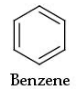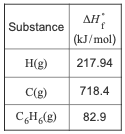# Problem: Part A. What is the standard enthalpy change for the reaction that represents breaking all the bonds in gaseous benzene, C6H6(g)? Express your answer to one decimal place and include the appropriate units.Determine the standard enthalpy change for the reaction that represents breaking all of the bonds in benzene, determine the equation for breaking all the carbon-carbon bonds of benzene use Hess's law to calculate the enthalpy change of this reaction and calculate the average bond enthalpy of a carbon-carbon bond in benzene This tutorial has been developed from Brown/LeMay/Bursten Integrative Exercise 8.105 and is a multiple-concept tutorial.Consider benzene in the gas phase, C6H6(g).Use the heat of formation, ΔH°f , values below to answer the questions.

###### FREE Expert Solution
83% (298 ratings)View Complete Written Solution
###### Problem Details

Part A. What is the standard enthalpy change for the reaction that represents breaking all the bonds in gaseous benzene, C6H6(g)?

Express your answer to one decimal place and include the appropriate units.

Determine the standard enthalpy change for the reaction that represents breaking all of the bonds in benzene, determine the equation for breaking all the carbon-carbon bonds of benzene use Hess's law to calculate the enthalpy change of this reaction and calculate the average bond enthalpy of a carbon-carbon bond in benzene This tutorial has been developed from Brown/LeMay/Bursten Integrative Exercise 8.105 and is a multiple-concept tutorial.

Consider benzene in the gas phase, C6H6(g).Use the heat of formation, ΔH°f , values below to answer the questions.